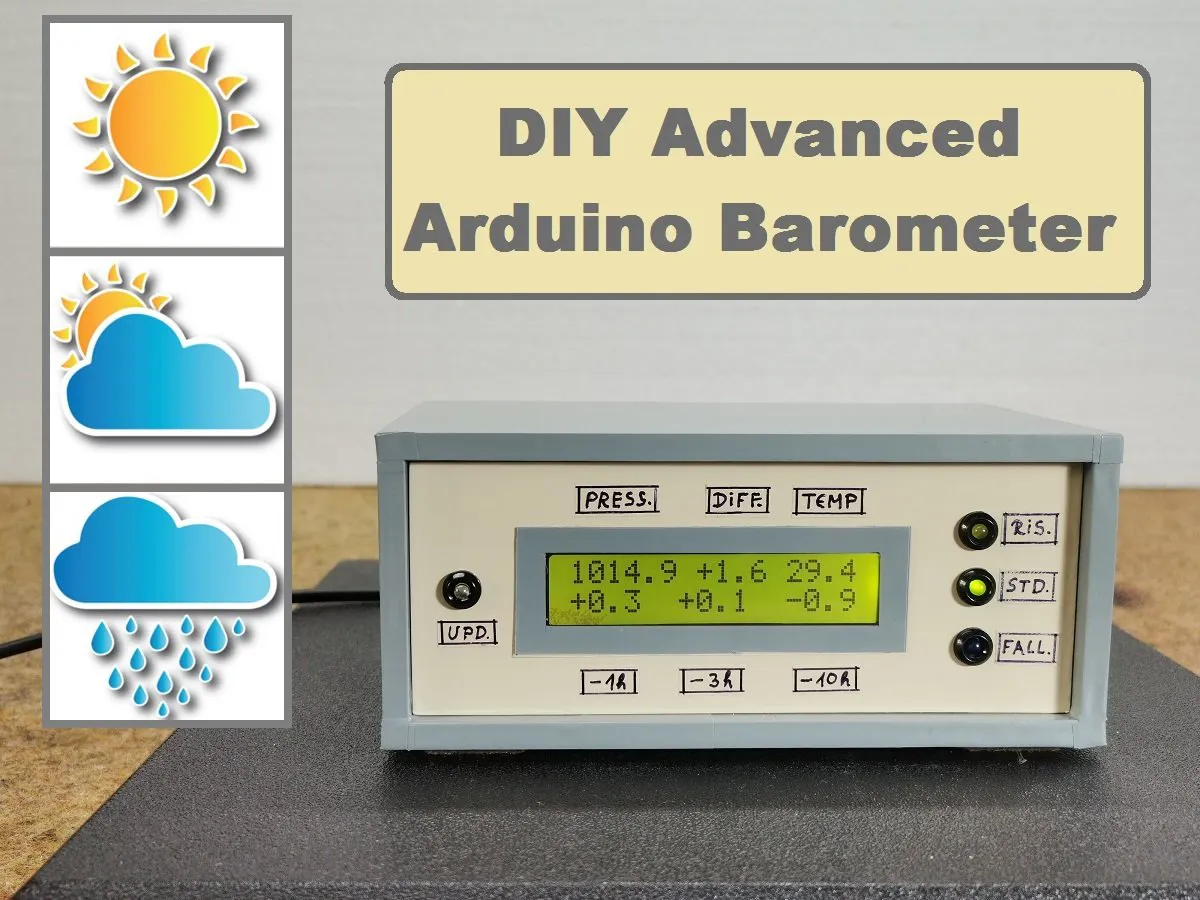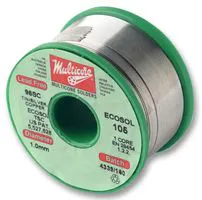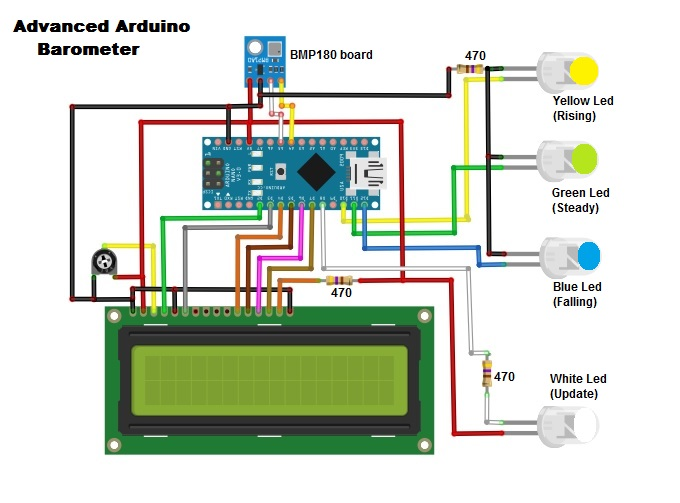Project tutorialPressure tendency can forecast short-term changes in the weather. This is a very sophisticated device designed for sailors, yachtsmen, etc.

• 2 views
• 0 respects

## Necessary tools and machinesSoldering iron (generic)## Apps and online services

A barometer is a scientific instrument that is used to measure atmospheric pressure. Pressure tendency can forecast short-term changes in the weather. This time I will show you how to make an advanced barometer.

Inexpensive home weather stations only show images of raindrops, clouds or the sun. More advanced weather stations show the current pressure value as a number, and the pressure change over the preceding few hours as a rough bar graph, mainly for decorative purposes. Such weather stations are significantly more expensive. Also on the market, there are very sophisticated devices designed for sailors, yachtsmen, etc., with high accuracy showing both pressure changes and the current value, but such devices are very expensive. The device I present to you has all the features of such expensive barometers, but it is very simple to build and the price is many times lower. The original project is described on the cxem.net website and I made some modifications to the code so that now the pressure values are displayed in Hectopascals, and there are also 3 LEDs to show the pressure trend.

It consists of only a few parts:

- Arduino Nano microcontroller

- BMP180 pressure sensor board, which is in direct contact with the surrounding air through this grille for more accurate temperature measurement

- LCD Display 16x2

- two resistors

- and four Leds

The measurement results are displayed on two lines. Immediately after turning on the barometer, the second line will be empty. Numerical values will appear there after 1 hour, 3 hours, and 10 hours, respectively. The first line displays the result of measuring the current atmospheric pressure in Hectopascals, next the deviation of the current pressure value from the average value for a given location, as well as the air temperature in degrees Celsius. The data shown in the top line is refreshed every 6 seconds, and this is displayed by a short flash of the white LED. The second line of the indicator displays the pressure increments over the last hour, three hours, and ten hours. If the pressure has increased during the specified time period, then the corresponding increment is displayed with a plus, otherwise - with a minus. The data in the second line is updated every 10 minutes. The three LEDs show the trend of Atmospheric pressure. If the pressure rises by more than 1.6 hectopascals in the last three hours, the yellow LED lights up (pressure rises). If the change is less than plus / minus 1.6 Hectopascals, the pressure is stable. And if the change is negative and greater than -1.6 hectopascals then we find that the pressure falling. These are quite a number of parameters based on which we can predict the local weather in the short term without using the Internet. Basically, the rising trend indicates a weather improvement, and a falling trend indicates a worsening. Standing pressure means stable weather.

Finally, the device is mounted in a suitable box made of PVC board and coated with self-adhesive wallpaper to get a professional look.

## Code

##### Arduino codeC/C++
```#include <Wire.h>
#include <LiquidCrystal.h>
LiquidCrystal lcd(2,3,4,5,6,7);

const float mid_p = 1013.25; // Sea level atmospheric pressure
int rLed = 10; // Rising Led
int sLed = 11; // Steady Led
int fLed = 12; //Falling Led
int i;                    // For loop counter
int j;                    // -------//---------
long p;                   // Current pressure in Pa (1Pa = 0.0075006376 mmHg)
long sum_p6;              // The sum of the results of pressure measurements for 6 sec.
long mini_p6;             // Minimum pressure in 6 seconds
long maxi_p6;             // Maximum pressure in 6 sec.
float  hg_p;              // Pressure in mmHg
float dif_pm;             // Difference of pressure - current and average (mid_p) in mm Hg.
float dif_p1;             // The difference in pressure - current and what was 1 hour ago
float dif_p3;             // The difference in pressure - current and what was 3 hours ago
float dif_p10;            // The difference in pressure - current and what was 10 hours ago
boolean flag;             // Flag allowing to output dif_p1, dif_p3, dif_p10 with two additional spaces
long sum_p;           // Array for storing 60 sums p. Each amount - 10,000 measurements in 10 minutes

float t;                  // Current temperature in degrees C
float sum_t6;             // The sum of the temperature measurement results in 6 seconds
float mini_t6;            // Minimum temperature in 6 sec
float maxi_t6;            // Maximum temperature in 6 sec

void setup() {
Serial.begin(9600);
pinMode (8, OUTPUT); // An LED is connected to D8 (11th leg) - a signaling device for the output of a new hg_P
pinMode(rLed,OUTPUT);
pinMode(sLed,OUTPUT);
pinMode(fLed,OUTPUT);
lcd.begin (16,2);

for(i=0; i<60; i++)               // Zeroing the array sum_p [i]
sum_p[i]=0;

if (!bmp.begin()) {               // Pressure sensor defective
lcd.print("BMP-180 DAMAGED!");
while (1) {}                      // Loop
}
}

void loop() {
sum_p = 0;                     // In the for loop for j in sum_p  we will accumulate the sum of 10,000 measurements
for (j=0;j<100;j++) {             // External cycle - we carry out 10,000 measurements in 600 seconds

sum_p6=0;                       // Preparing data for the inner loop
mini_p6=110000;                 // Knowingly more than possible
maxi_p6= 80000;                 // Deliberately less than possible
sum_t6=0;
mini_t6=100.0;                  // Knowingly more than possible
maxi_t6=0.0;                    // Deliberately less than possible

for(i=0;i<102;i++) {          // Internal loop - we carry out 102 measurements in 6 seconds

Serial.println(p);
if (p<mini_p6)              // Find the max and min results to discard later
mini_p6=p;
if (p>maxi_p6)
maxi_p6=p;
sum_p6=sum_p6 + p;

if (t<mini_t6)
mini_t6=t;
if (t>maxi_t6)
maxi_t6=t;
sum_t6=sum_t6 + t;

delay(18);                  // Adjusting the duration of the inner loop to 6 seconds
}                             // finished an internal 6-second loop on i

sum_p=sum_p+sum_p6-maxi_p6-mini_p6;
hg_p=1.0E-4 * (sum_p6-maxi_p6-mini_p6) + 77.32;  // 77.332 - Difference between Absolute, and Relative pressure for a given place
dif_pm=(hg_p - mid_p);
digitalWrite (8,LOW);           // We turn on the LED - display a new result

// We display the top line on the indicator - current pressure, deviation_
// average pressure and temperature.

lcd.home();
lcd.print (hg_p,1);
lcd.print (" ");
if (dif_pm >=0)                 // Знак "+" или "-" ставим сами
lcd.print ("+");
else
lcd.print ("-");
lcd.print (abs (dif_pm),1);
lcd.print (" ");
if (abs(dif_pm)>=9.95){         // If dif_pm is long (5 familiar spaces), then t is displayed without tenths
lcd.print (0.01*(sum_t6-maxi_t6-mini_t6),0);
lcd.print (" ");              // Clearing the 16th digit
}
else                            // Otherwise, we print t with tenths
lcd.print(0.01*(sum_t6-maxi_t6-mini_t6),1);

delay(83);                      // Adjusting the duration of the outer cycle to 10 minutes
digitalWrite (8,HIGH);          // Turning off the LED

}                                 // finished an outer 10-minute cycle on j

dif_p1 = (sum_p - sum_p) * 1.0E-6;
dif_p3 = (sum_p - sum_p)* 1.0E-6 ;
dif_p10 = (sum_p - sum_p)* 1.0E-6 ;

flag=false;                     // no spaces can be inserted into the 2nd line
if (abs(dif_p1)<9.95 and abs(dif_p3)<9.95 and abs(dif_p10)<9.95)
flag=true;                    // If dif_p occupy 4 familiar spaces, then spaces can be inserted
if (sum_p==0)               // If there is no data for dif_p10 yet, then spaces can be inserted
flag=true;

// We output dif_p1, dif_p3 and dif_p10 to the 2nd (bottom) line of the indicator

lcd.setCursor (0,1);
lcd.print ("                "); // Clearing the 2nd line
lcd.setCursor (0,1);

// Час назад...

if (sum_p>0) {               // If data collection started more than an hour ago
if (dif_p1 >=0)               // We put the "+" or "-" sign ourselves
lcd.print ("+");
else
lcd.print ("-");
lcd.print (abs (dif_p1),1);
lcd.print (" ");
}
else                            // No data yet ...
lcd.print ("--.- ");

if (flag==true)
lcd.print (" ");

// 3 Часа назад...

if (sum_p>0) {              // If data collection started more than 3 hours ago
if (dif_p3 >=0)               // We put the "+" or "-" sign ourselves
lcd.print ("+");
else
lcd.print ("-");
lcd.print (abs (dif_p3),1);
lcd.print (" ");
}
else                            // No data yet ...
lcd.print ("--.- ");

if (flag==true)
lcd.print (" ");

// 10 Часов назад...

if (sum_p>0) {              // If data collection started more than 10 hours ago
if (dif_p10>=0)               // We put the "+" or "-" sign ourselves
lcd.print ("+");
else
lcd.print ("-");
lcd.print (abs (dif_p10),1);
}
else                            // No data yet ...
lcd.print ("--.-");

// Shift the contents of the sum_p [] array one position to the right

for(i=59;i>0;i--)
sum_p[i] = sum_p[i-1];

if (dif_p3 > 1.6) {
digitalWrite(rLed, HIGH);
digitalWrite(sLed, LOW);
digitalWrite(fLed, LOW);
}
if (dif_p3 < -1.6) {
digitalWrite(rLed, LOW);
digitalWrite(sLed, LOW);
digitalWrite(fLed, HIGH);
}
if (dif_p3 > -1.6 && dif_p3 < 1.6) {
digitalWrite(rLed, LOW);
digitalWrite(sLed, HIGH);
digitalWrite(fLed, LOW);
}
}

// End of sketch
```

## Schematics#### DIY Simple Weather Forecast Device

Project tutorial by Mirko Pavleski

• 5,961 views
• 8 respects

#### Barometer with Analog Scale (Ammeter)

Project tutorial by Mirko Pavleski

• 3,162 views
• 9 respects

#### How to Make a Simplest Variometer for Paragliding

Project tutorial by Mirko Pavleski

• 3,711 views
• 4 respects

#### DIY Arduino Height Measuring Machine

Project tutorial by Mirko Pavleski

• 14,027 views
• 37 respects

#### DIY Simple Autorange Capacitance Meter (10pF-10000microF)

Project tutorial by Mirko Pavleski

• 14,584 views